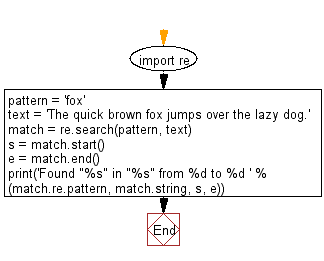﻿ Python Exercises: Search a literals string in a string and also find the location where the pattern occurs - w3resource# Python: Search a literals string in a string and also find the location where the pattern occurs

## Python Regular Expression: Exercise-20 with Solution

Write a Python program to search a literals string in a string and also find the location within the original string where the pattern occurs.

Sample Solution:-

Python Code:

``````import re
pattern = 'fox'
text = 'The quick brown fox jumps over the lazy dog.'
match = re.search(pattern, text)
s = match.start()
e = match.end()
print('Found "%s" in "%s" from %d to %d ' % \
(match.re.pattern, match.string, s, e))
```
```

Sample Output:

```Found "fox" in "The quick brown fox jumps over the lazy dog." from 16 to 19
```

Pictorial Presentation:Flowchart:## Visualize Python code execution:

The following tool visualize what the computer is doing step-by-step as it executes the said program:

Python Code Editor:

Have another way to solve this solution? Contribute your code (and comments) through Disqus.

What is the difficulty level of this exercise?

Test your Python skills with w3resource's quiz

﻿

## Python: Tips of the Day

Merges two or more lists into a list of lists, combining elements from each of the input lists based on their positions

Example:

```def tips_merge(*args, fill_value=None):
max_length = max([len(lst) for lst in args])
result = []
for i in range(max_length):
result.append([
args[k][i] if i < len(args[k])
else fill_value for k in range(len(args))
])
return result
print(tips_merge(['x', 'y'], [1, 2], [True, False]))
print(tips_merge(['x'], [1, 2], [True, False]))
print(tips_merge(['x'], [1, 2], [True, False], fill_value = '_'))
```

Output:

```[['x', 1, True], ['y', 2, False]]
[['x', 1, True], [None, 2, False]]
[['x', 1, True], ['_', 2, False]]
```